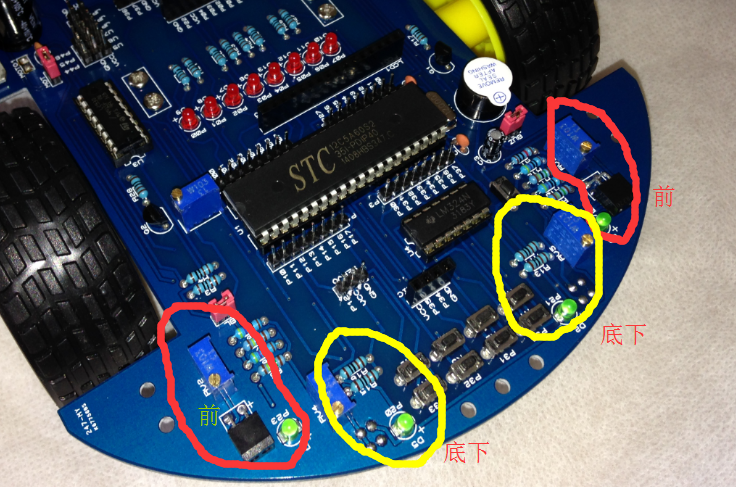# 智能小车搭建(3)–红外传感器#include "STC12C5A60S2.h"
#include <intrins.h>

/**
红外传感器
**/
sbit p20 = P2^0; // 正极发射 0
sbit p23 = P2^3;// 	正极接收
sbit p24 = P2^4;
sbit p21 = P2^1;

sbit led0 = P0 ^ 0;
sbit led1 = P0 ^ 1;

void delay10ms(void)   //误差 -0.000000000001us
{
unsigned char a,b,c;
for(c=199;c>0;c--)
for(b=120;b>0;b--)
for(a=1;a>0;a--);
}
void delay500ms(void)   //误差 -0.000000000028us
{
unsigned char a,b,c;
for(c=71;c>0;c--)
for(b=168;b>0;b--)
for(a=250;a>0;a--);
}

/**
有障碍物 管脚为1  指示灯灭		RPR220D红外传感器输出1
无障碍物 管脚为0  指示灯亮		RPR220D红外传感器输出0
*/

void main()
{

while(1)
{
if(p23 == 0) {
led0 = ~led0;
led1 = 1;
delay500ms();
}
else {
led1 = 0;
}
}
}